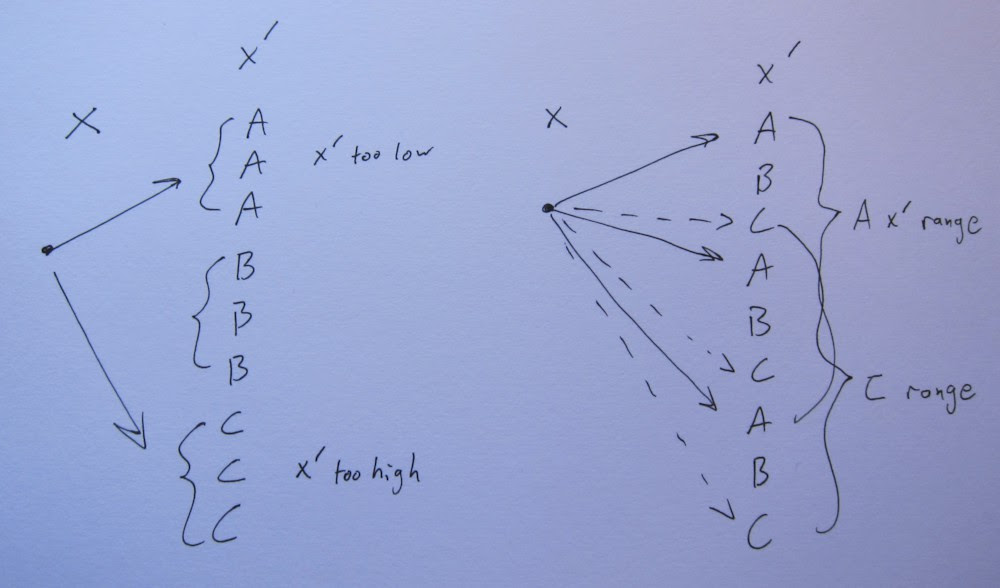cbloom rants

2/03/2014

02-03-14 - Understanding ANS - 5

First in case you aren't following them already, you should follow along with ryg and Yann as we all go through this :
RealTime Data Compression A comparison of Arithmetic Encoding with FSE
rANS notes The ryg blog

Getting back to my slow exposition track.

We talked before about how strings like "ABC" specify an ANS state machine. The string is the symbols that should be output by the decoder in each state, and there's an implicit cyclic repeat, so "ABC" means "ABCABC..". The cyclic repeat corresponds to only using some modulo of state in the decoder output.

Simple enumerations of the alphabet (like "ABC") are just flat codes. We saw before that power of two binary-distributed strings like "ABAC" are Huffman codes.

What about something like "AAB" ? State 0 and 1 both output an A. State 2 outputs a B. That means A should have twice the probability of B.

How do we encode a state like that? Putting in a B is obvious, we need to make the bottom of state be a 2 (mod 3) :

x' = x*3 + 2

but to encode an A, if we just did the naive op :

x' = x*3 + 0
or
x' = x*3 + 1

we're wasting a value. Either a 0 or 1 at the bottom would produce an A, so we have a free bit there. We need to make use of that bit or we are wasting code space. So we need to find a random bit to transmit to make use of that freedom. Fortunately, we have a value sitting around that needs to be transmitted that we can pack into that bit - x!

take a bit off x :
b = x&1
x >>= 1

x' = x*3 + b

or :

x' = (x/2)*3 + (x%2)

more generally if the output string is of length M and symbol s occurs Fs times, you would do :

x' = (x/Fs)*M + (x%Fs) + Bs

which is the formula for rANS.

Now, note that rANS always makes output strings where the symbols are not interleaved. That is, it can make "AAB" but it can't make "ABA". The states that output the same symbol are in consecutive blocks of length Fs.

This is actually not what we want, it's an approximation in rANS.

For example, consider a 3-letter alphabet and M=6. rANS corresponds to an output string of "AABBCC". We'd prefer "ABCABC". To see why, recall the arithmetic coding formula that we wanted to use :

x' = (x/P) + C

the important part being the (x/P). We want x to grow by that amount, because that's what gives us compression to the entropy. If x grows by too much or too little, we aren't coding with codelens that match the probabilities, so there will be some loss.

P = F/M , and we will assume for now that the probabilities are such that the rational expression F/M is the true probability. What we want is to do :

x' = (x*M)/F + C

to get a more accurate scaling of x. But we can't do that because in general that division will cause x' to not fall in the bucket [Cs,Cs+1) , which would make decoding impossible.

So instead, in rANS we did :

x' = floor(x/F)*M + C + (x%F)

the key part here being that we had to do floor(x/F) instead of (x/P), which means the bottom bits of x are not contributing to the 1/P scaling the way we want them to.

eg.

x = 7
F = 2
M = 6
P = 1/3

should scale like

x -> x/P = 21

x -> (x/F)*M + (x%F) = (7/2)*6 + (7%2) = 3*6 + 1 = 19

too low
because we lost the bottom bit of 7 when we did (7/2)

In practice this does in fact make a difference when the state value (x) is small. When x is generally large (vs. M), then (x/F) is a good approximation of the correct scaling. The closer x is to M, the worse the approximation.

In practice with rANS, you should use something like x in 32-bits and M < 16-bits, so you have decent resolution. For tANS we will be using much lower state values, so getting this right is important.

As a concrete example :

alphabet = 3
1000 random symbols coded
H = 1.585
K = 6
6 <= x < 12

output string "ABCABC"
wrote 1608 bits

output string "AABBCC"
wrote 1690 bits

And a drawing of what's happening :I like the way Jarek Duda called rANS the "range coder" variant of ANS. While the analogy is not perfect, there is a similarity in the way it approximates the ideal scaling and gains speed.

The crucial difference between a "range coder" and prior (more accurate) arithmetic coders is that the range coder does a floor divide :

range coder :

symhigh * (range / symtot)

CACM (and such) :

(symhigh * range) / symtot

this approximation is good as long as range is large and symtot is small, just like with rANS.

Anonymous said...

>When x is generally large (vs. M), then (x/F) is a good approximation of the correct scaling. The closer x is to M, the worse the approximation.

probably we can change formula
(x/F)*M + (x%F) -> (x*M)/F+(x*M)%F

Anonymous said...

probably not :(

Anonymous said...

I wouldn't be surprised if this was obvious to everyone else, but it took me a while to figure out that Bs seems to mean F1+...+F(s-1). Thanks for this nice series. It has helped me understand ANS.